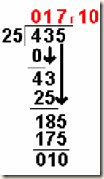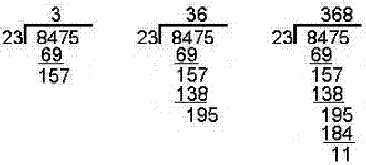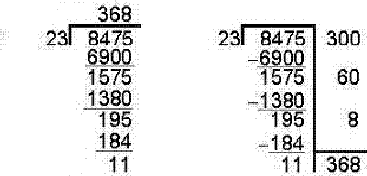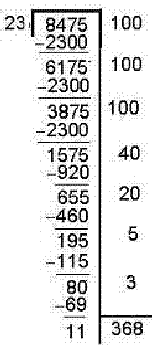## Friday, 14 October 2011

"As you become old, you will definitely forget your primary school rhymes!!.. similar to that, you would also forgot multiplication and division as well!!.. This is the dummy’s way to understand, Division with reminder.. Take 1 in the hand, 5 in the middle.. :)”

Introduction

Suddenly, i found that i forgot the 5th Grade Long division method. I don’t know about you. But thought of covering it, as its not so simple.. :)

It’s not a pure dummies or a 5th grade guide. We need to understand Long division method clearly to go in-depth for doing Division of Large numbers or Getting reminder between two large numbers.

As you might guessed, division of large numbers (as heavy as 2048-bit size) are used now in RSA which is also proven to be cracked within years!!.. :)

As this method is required basics for RSA encryption algorithm, this article will be useful for a computer geek as well as a 5th grader!!..

Concept

The way we do long division is simple. But underlying logic behind division is very important one to understand. Multiplication if you take,

how we come up with 4*3 = 12 ? 4 + 4 + 4 or 4 added 3-times gives you 12. So for a successful multiplication, just successive additions are more than enough. Even in current systems, they just use the adder circuit at ALU to do this.

Then what does 12/3 does ? There are 2-types are division a system categorized into,

• integer division
• floating point division

12/3 will yield you just an integer. So, based on programming language and system, it selects to use integer division where floating point is completely ignored.

Try, int z = 12/5

You will just get z=2. At the same case, if you convert these to float, you get the mantissa & exponential part which might require more than 32-bit. (float is just 32-bit, double is 64-bit).

Try this now,     float z=12.0/5.0;

The above is an example of floating point division.

At the core, division happens using subtraction. 12/3 means.. how many times subtracting three yields zero.

“Ok, i understood int, float. What is quotient and reminder ?”

(Take example: 12/3) - quotient(4) is the number you get after you divide with divisor (3) the dividend(12). Sometimes, just subtracting denominator with numerator will not yield zero. Subtract as much as you can so that, the value is less than your quotient. This value will become your reminder.If you want to continue subtracting, then it must be after a decimal point.In the above division, 10 is the reminder and 17 is the value you get in integer division. now to find the exponential part, you can further divide from there, 10/25.. :) you get 0.4. So the resultant of this division is 17.4.

More explanation

Division is just a concept of finding out how many values are inside a value

Just take the below long division example,This is the normal way, we have learnt the division. But actually what happens here is different and this needs to be understood to write code to do large number division.The above is the right way our teachers should have explained. We don’t just take the first 2 digits, we always assume that we have 0’s to complement as. 23*3 = 69 if we do and just subtract the first 2 digit simply means that there are 0’s in the other places. we have to finally add, all these 300 + 60 + 8 to get the actual quotient. Remember that, 11 is the reminder we have got. If we further divide, 11/23.. you get decimal pointed values.Thus, it doesn’t mean that you need to always have 23*3 for your operation. you can even do like the above way, if you want to step in a easy multiplicative way.

References

http://en.wikipedia.org/wiki/Binary_multiplier

http://www.conceptualstudy.org/Elementary%20Math/Understanding%20Division.htm

Some exercises

“Now, how would you find the reminder between 56.74 / 45.67647”

In C++, you have fmod. Normally, you can do the following,

• first do integer division of the above numbers - 56/45
• now to get the reminder do this, 56.74 – 45.67647 * quotient (which is 1 in our case)
• this gives out our reminder as, 11.06